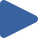# Heap Sort

## Animation, code, analysis, and discussion of heap sort on 4 initial conditions.

How to use: Press "Play all", or choose thebutton.

 Play All Play animationRandomPlay animationNearly SortedPlay animationReversedPlay animationFew Unique### ALGORITHM

``````# heapify
for i = n/2:1, sink(a,i,n)
→ invariant: a[1,n] in heap order

# sortdown
for i = 1:n,
swap a[1,n-i+1]
sink(a,1,n-i)
→ invariant: a[n-i+1,n] in final position
end

# sink from i in a[1..n]
function sink(a,i,n):
# {lc,rc,mc} = {left,right,max} child index
lc = 2*i
if lc > n, return # no children
rc = lc + 1
mc = (rc > n) ? lc : (a[lc] > a[rc]) ? lc : rc
if a[i] >= a[mc], return # heap ordered
swap a[i,mc]
sink(a,mc,n)
``````

### DISCUSSION

Heap sort is simple to implement, performs an O(n·lg(n)) in-place sort, but is not stable.

The first loop, the Θ(n) “heapify” phase, puts the array into heap order. The second loop, the O(n·lg(n)) “sortdown” phase, repeatedly extracts the maximum and restores heap order.

The sink function is written recursively for clarity. Thus, as shown, the code requires Θ(lg(n)) space for the recursive call stack. However, the tail recursion in sink() is easily converted to iteration, which yields the O(1) space bound.

Both phases are slightly adaptive, though not in any particularly useful manner. In the nearly sorted case, the heapify phase destroys the original order. In the reversed case, the heapify phase is as fast as possible since the array starts in heap order, but then the sortdown phase is typical. In the few unique keys case, there is some speedup but not as much as in shell sort or 3-way quicksort.

### KEY

• Black values are sorted.
• Gray values are unsorted.
• A red triangle marks the algorithm position.

### PROPERTIES

• Not stable
• O(1) extra space (see discussion)
• O(n·lg(n)) time